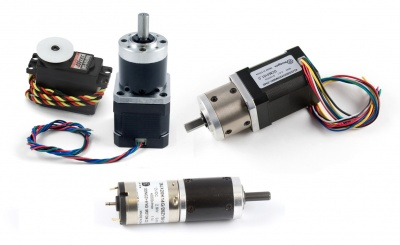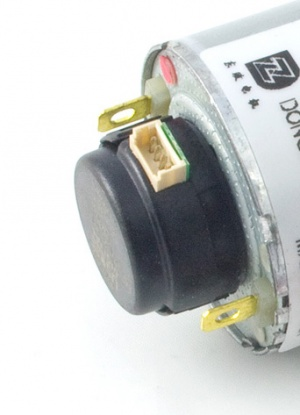Motor Selection Guide

Choosing a MotorA servo motor (left), a stepper motor (middle), a BLDC motor (right) and a DC motor (bottom).

There are 4 categories of electric motors that are used in practical applications and are easily available for purchase. They are:

Each of these classes has several variants and each have their advantages and disadvantages.

There are many different options when considering what type of motor to use. This table provides a summary of the differences:

 Motor Type Rotation Position Control Velocity Control Position Precision Price Range Controller Price per Motor Brushed DC Motors Continuous None Open-Loop N/A $10+$60+ Brushed DC Motors (w/ Encoder) Continuous Closed-Loop Closed-Loop Varies1 $40+$60+ Servo Motors Limited (Usually °180) Closed Loop Closed Loop 0.5° - 1.5° $12+$6+ Continuous Rotation RC Servo Motors Continuous None Open Loop N/A $18+$6+ Stepper Motors Continuous Open Loop Open Loop 0.9°-1.8° (or 1°-3° with gearbox)2 $15+$70+ Stepper Motors (w/Encoder) Continuous Closed Loop Closed Loop 0.9°-1.8° (or 1°-3° with gearbox)2 $25+$85+ Brushless DC Motors Continuous Closed Loop Closed Loop Varies3 $35+$65+

1- The positional precision of a DC motor with encoder depends on the encoders CPR (counts per revolution). For example, a 360 CPR encoder would provide 1° precision.

2- Gearboxes introduce inaccuracy in the form of "slop" (gap between mated gear teeth). For maximum precision, stick with gearless steppers with 0.9° step angle.

3- The positional precision of a BLDC motor varies depending on the hall effect sensor in the motor and how the controller measures the data from it.

Rotation

While most motors have fully continuous rotation, there is one type of motor that has limited rotation: servo motors. Most servos have 180° of travel, but you can find some multi-rotation servos designed to be part of a winch mechanism. The limited rotation angle of servo motors is a tradeoff for having built-in position control using a potentiometer.

Position Control

If you want to be able to specify a precise position for your motor to rotate to and stop, you'll need some form of position control. There are two main types of control.

• Open-Loop position control means the motor controller has a way of telling the motor to move to a certain position, but there's no way for the controller to know if the motor actually succeeded in reaching that position. For example, if the motor stalled because it came in contact with a solid object, the controller would have no way of knowing this. Open-loop control is sufficient in systems where such external forces are limited, or where exact positioning is not critical.
• Closed-Loop position control has some sort of feedback mechanism so that the controller knows the position of the motor at all times. This means that even if an external force resists or opposes the motor's movement, the controller will know to keep driving the motor until it reaches its target position. Closed-loop position control is required in systems where positioning errors are not acceptable, or in systems that run for long periods of time that could otherwise accumulate positioning errors during operation.

Motor Comparison:

• BLDC motors and use hall-effect sensors built into the motor for feedback, allowing for closed-loop control.
• RC Servos have closed-loop position control because servo controllers read the built-in potentiometer of the servo for feedback.
• Stepper motors have open-loop control, since the controller tells the motor to move a certain number of steps.
• DC Motors do not have any position control, as the controller simply decides how much power to provide to the motor, which determines motor speed.

Systems without closed-loop position control can be upgraded by adding an encoder to the motor. Combined with a device that can read it, the encoder will provide feedback that will enable closed-loop control.

Velocity Control

A system can also have velocity control, which allows it to target a specific rotation speed. If your system has open-loop position control, it will (by definition) have open-loop velocity control. Likewise, a system with closed-loop position control will have closed-loop velocity control.

• DC Motors and continuous rotation servos both have open-loop velocity control, because the controller simply decides how much power to apply to the motor and this influences the speed directly.
• Stepper motors have open-loop velocity control because the controller knows the desired position and the amount of time it takes to send the signal to get to that position, so velocity can easily be calculated.
• BLDC Motors have closed-loop velocity control, since the controller knows the position and timing due to data from the hall effect sensors in the motor.
• Limited rotation servos have closed-loop velocity control, since the controller knows the position and timing due to data from the potentiometer.

In the same way that encoders can be used to upgrade the position control of a system, they can also provide closed-loop velocity control.

Precision

There are a number of factors that impact the precision of a motor's position control, depending on the type of motor and what kind of feedback mechanism it uses.

Stepper motors are accurate to whatever the motor step angle is. The typical step angle is 1.8°, in which case the motor's positioning would always be a multiple of 1.8°, resulting in 200 unique positions across a full rotation.

Servo motors are limited by the "Deadband width", which can be found in your servo motor's data sheet. Take the full pulse range of the servo and divide it by the deadband width. Then take the servo's actuation range in degrees and divide it by the result. This will give you the servo's position resolution in degrees.

If you're using an encoder, the precision will be limited by the encoder's CPR. For example, a CPR of 360 will result in 1° resolution.

If your motor has a gearbox, it will introduce some amount of error in positioning due to empty space between mated gear teeth. This is called "backlash" or "slop". Typical gearboxes can have anywhere from 1 to 3 degrees of slop, which impacts your positioning precision directly.

Price Range

Due to differences in how motors are built and controlled, the prices between types of motor vary drastically.

Servo motors are often the most economical option for low-torque applications. While the price for a single motor is comparable to other options, servo controller boards are often set up to control as many as 16 servos at once.

DC motors are the simplest type of motor, and are available in a wide range of sizes. DC controllers are usually fairly simple, so the controller cost isn't as high as steppers or BLDC.

Stepper motors and BLDC motors are more expensive, because the motors as more complicated to control.

Gearboxes

Gearboxes, a common feature of electric motors, use the mechanical advantage of gears to reduce the speed of the motor and increase the torque. The speed and accuracy are affected directly by the gear ratio, as seen in these equations:

${\text{Output Speed}}={\frac {\text{Motor Speed}}{\text{Gearbox Ratio}}}$

For example, a motor with a speed of 500 rpm and a gearbox with a 10:1 gear ratio would result in and output speed of 50 rpm.

Although the reduction ratio plays a large part in determining the Gearbox Output Torque, there is also an inefficiency that is introduced through the use of a gearbox. Some of the torque of the motor is converted into heat and lost due to friction between the gears. Gearbox efficiency depends on the physical characteristics of the gears, the number of gear stages, and gearing system used. You can calculate the Gearbox Output Torque with the following equation:

${\text{Output Torque}}={\text{Motor Output Torque }}\times {\text{ Gearbox Ratio }}\times {\text{ Gearbox Efficiency}}$

Keep in mind that while adding a gearbox does increase the torque, the gearbox itself is only rated to withstand a certain amount of torque before it risks becoming damaged or breaking. When selecting a motor, you should always consider the actual operating torque to be the minimum of the output torque and the gearbox strength. For example, a motor that can normally output 5 kg·cm of torque with a 10:1, 90% efficient gearbox whose gears are rated for 40 kg·cm would theoretically be able to output 45 kg·cm of torque, but in practice the 40 kg·cm limit of the gearbox should be considered the maximum torque.

With gearboxes, torque and speed can be seen as one interchangeable characteristic: If you need more torque and less speed, try to find the same motor with a gearbox with a higher reduction ratio. If you need more speed and less torque, try to find the same motor with a gearbox with a lower reduction ratio. However, it is not advisable buy gearboxes and motors separately to mix and match, unless they are specifically designed for each other. There's a lot that can go wrong in gearbox customization and for most users it's a lot less hassle to simply buy a motor with a gearbox already attached.

EncodersA small optical encoder mounted on the rear shaft of a DC motor.

An encoder is a device installed on the shaft of a motor that can be used to keep track of the position of the motor or calculate the speed of the motor. Encoders are often used with a DC motor and a PID control system in order to achieve position/velocity control of a motor that would otherwise only have simple on/off control. Some motors have the shaft exposed on both sides of the motor, so an encoder can be attached on one end without getting in the way of what the motor is driving.

Encoders are rarely used with BLDC motors or servo motors, since they both already have some form of closed-loop position feedback built-in. Encoders are sometimes used with stepper motors, because position control in a stepper motor is open-loop (the controller has no way of knowing whether the stepper actually made it to the desired position, it only knows how far it has told the stepper to go). Excessive vibration, external forces, or sudden high-torque loads can cause steps to be missed, so an encoder would close the control loop and provide actual feedback in this case.

Estimating Required Torque

It can be difficult to estimate how much torque will be required for a certain application before the project is built, but there are plenty of guidelines and rules-of-thumb online that can give you a good idea.

In the case of a typical wheeled vehicle, we recommend you take the mass of the vehicle and multiply by the radius of the wheels, then divide by four.

${\text{Estimated Torque Requirement}}={\frac {{\text{Mass }}\times {\text{ Wheel Radius }}}{4}}$

For example, a 20kg vehicle with wheels of radius 6cm would call for a total goal of 30 kg·cm contributed from all of the motors. This should be more than enough torque to move around on smooth terrain and gentle inclines. If you need to be able to handle rough terrain or sharp inclines, you may want to only divide by a factor of 3 or even 2. In general, it's a good idea to get a more powerful motor than you think you'll need, just to account for unforeseen factors.